Area of combination of figures - two circles, circle and square

Chapter 9 Class 7 Perimeter and Area
Concept wise

Let’s take a rectangle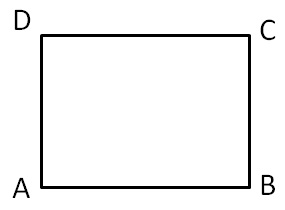And add a semicircle on top of side CD & AB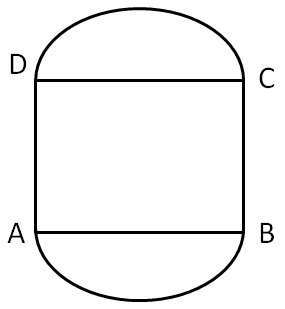#### What will be the area of total figure?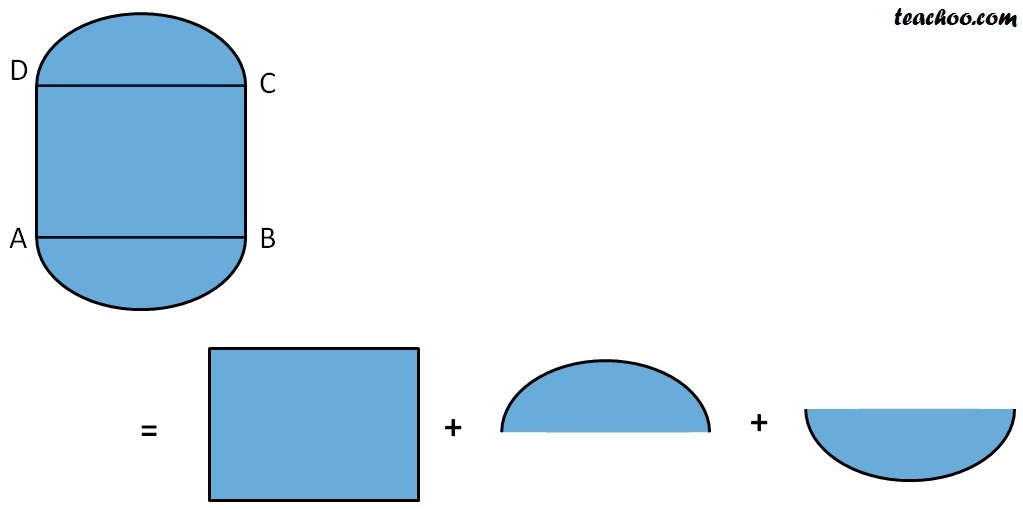Area of total figure = Area of rectangle + Area of top semicircle + Area of bottom semicircle.

Let’s take some values & actually solve it

What will be the area of the figure given below?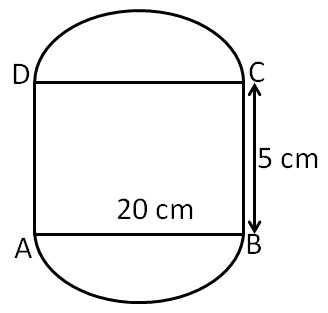Here,

Area of total Figure =  Area of rectangle  + Area of top semicircle + Area of bottom semicircle

Here, Both semicircle will have the same diameter,

So, their area will be the same

∴ Area of total figure = Area of rectangle + 2 × Area of semicircle

Let’s find them out separately

#### Area of rectangle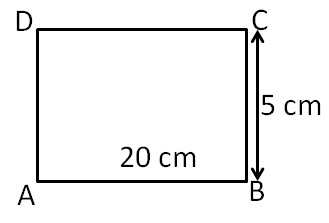Length = l = AB

= 20 cm

= 5 cm

Area of rectangle ABCD = l × b

= AB × BC

= 20 × 5

= 100 cm 2

#### Area of semi-circle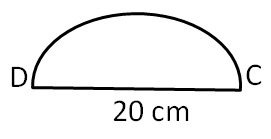Diameter = 20 cm

= 20/2

= 10 cm

Now,

Area of semi-circle = (Area of circle)/2

= πr 2 /2

= (3.14  (10) 2 )/2

= (314 × 100)/(100 × 2)

= 314/2

= 157 cm 2

Now,

Area of figure = Area of rectangle ABCD + 2 Area of semi-circle

= 100 + 2 × (157)

= 100 + 314

= 414 cm 2

∴ Area of given figure is 514 cm 2

Learn in your speed, with individual attention - Teachoo Maths 1-on-1 Class